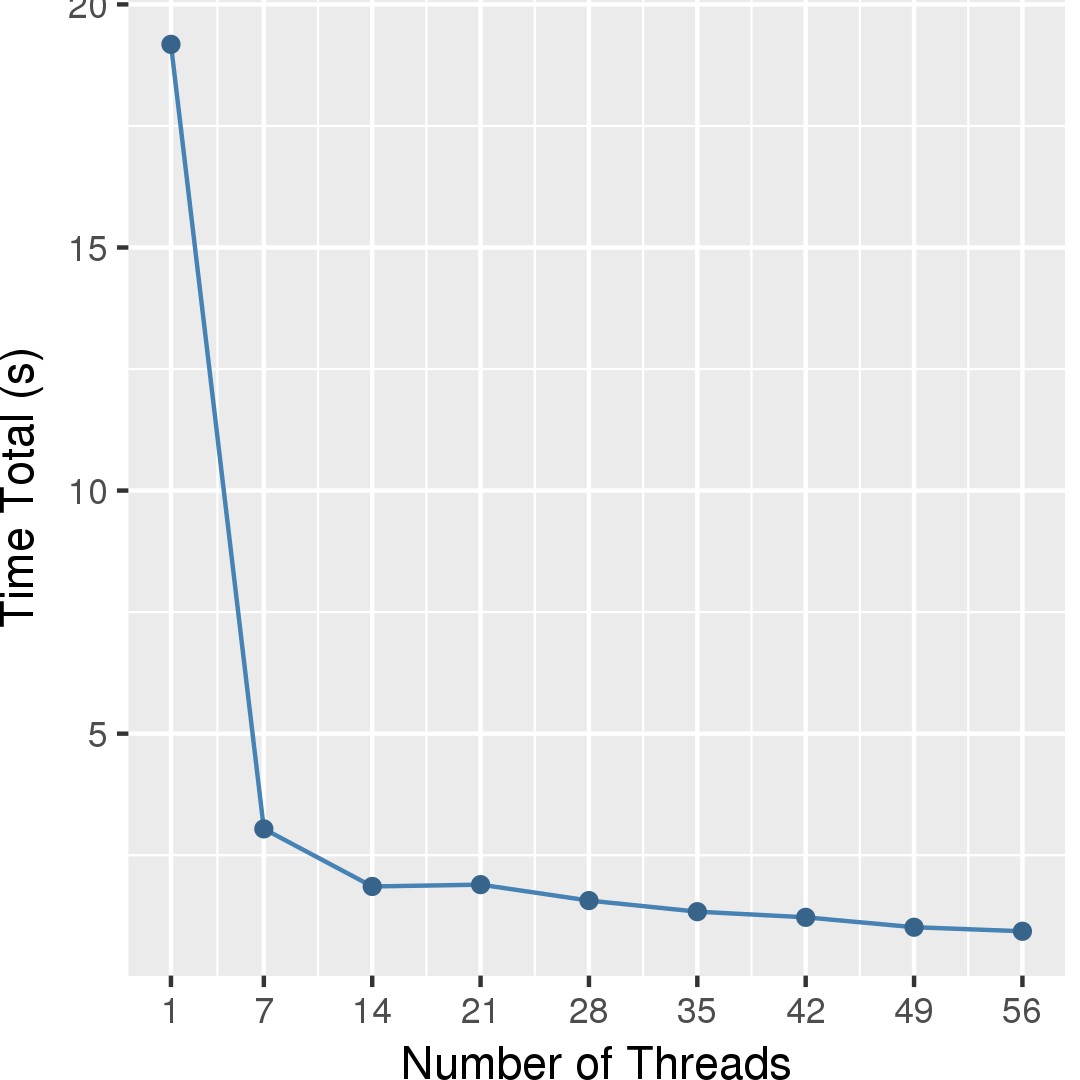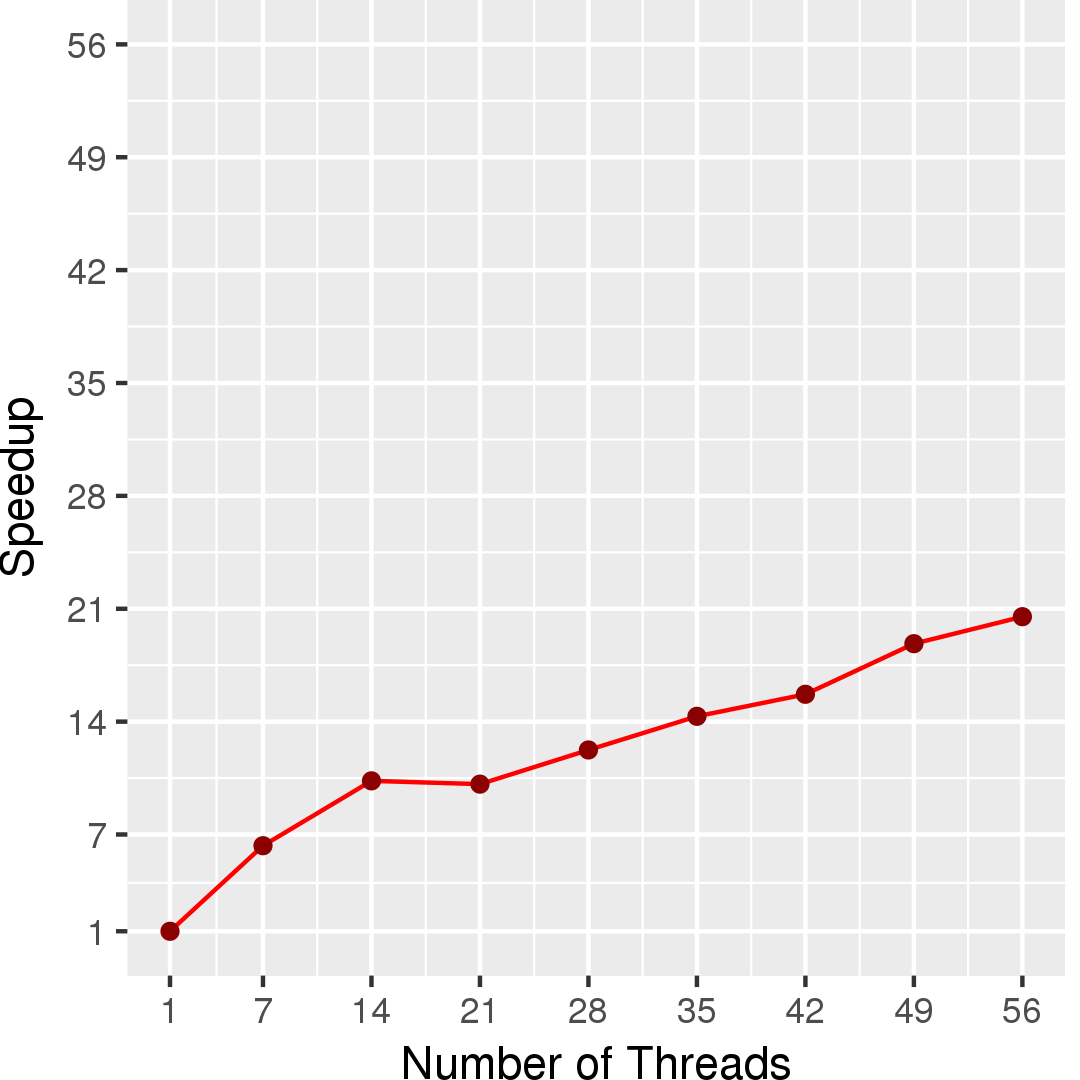## Application Description

This benchmark computes the shortest path from a source node to all nodes in a directed, unweighted graph by using a modified Bellman-Ford algorithm .

## Algorithm

This algorithm computes the solution to the single-source shortest path problem with unit edge weights using a demand-driven modification of the Bellman-Ford algorithm. Each node maintains an estimate of its shortest distance from the source called dist. Initially, this value is infinity for all nodes except for the source, whose distance is 0. The algorithm proceeds by iteratively updating distance estimates starting from the source and maintaining a worklist of nodes whose distances have changed and thus may cause other distances to be updated. Figure 1 gives the pseudocode for the algorithm.

In principle, one can directly apply any general single-source shortests paths (SSSP) algorithm to solve this problem, but there are some optimizations that are useful to apply in this specific context.

The general SSSP algorithm above may add multiple items to the worklist that update the same node to the same distance value (so-called empty work). The amount of empty work can be quite large, so it pays to avoid adding it if possible. The algorithm in Figure 1 does this by first checking if a node needs to be added before adding it to the worklist (line 10). For simplicity, the general SSSP algorithm above skips this step.

If the scheduler always processes all the work at the current distance level before moving on to the next distance (i.e., bulk-synchronous scheduling), updates to the distance of neighbors can be made without synchronization because all updates within a distance level will be the same.

 1 2 3 4 5 6 7 8 9 10 11 Graph g = /* read input */; WorkList frontier = new WorkList(); source.dist = 0; frontier.add(source); foreach Vertex c : frontier do foreach Vertex n : c.getNeighbors() do if (n.dist <= c.dist) continue; n.dist = c.dist + 1; frontier.add(n); end for end for

Figure 1: Pseudocode for breadth-first search algorithm.

## Data Structures

There are two key data structures used in this algorithm:

Unordered Set
The worklist containing the items that need to be processed is an unordered set (since items can be processed in any order).
Directed Graph

## Caveats

This implementation breaks from the Galois model by atomically updating the distance value in nodes rather than taking a lock on the node, and when bulk-synchronous scheduling is used, distance values are updated without any synchronization.

## Performance

Figure 2 and Figure 3 show the execution time in seconds and self-relative speedup (speedup with respect to the single thread performance), respectively, of this algorithm running on the Twitter graph.## Machine Description

Performance numbers are collected on a 4 package (14 cores per package) Intel(R) Xeon(R) Gold 5120 CPU machine at 2.20GHz from 1 thread to 56 threads in increments of 7 threads. The machine has 192GB of RAM. The operating system is CentOS Linux release 7.5.1804. All runs of the Galois benchmarks used gcc/g++ 7.2 to compile.